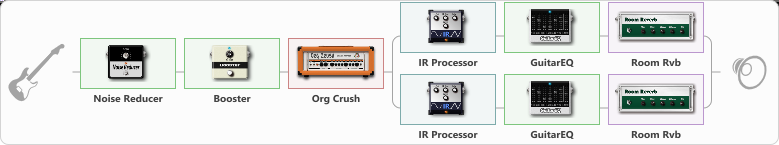# Rustam

Discussion in 'ToneLib-GFX presets' started by Assymmetry, Mar 6, 2023.

1. Rustam

Effects chain:Effect: "Noise Reducer" (Dynamics / Filter), active - "yes"
{
"Sens" = 100
"Mode" = Soft
}

Effect: "Booster" (Dynamics / Filter), active - "yes"
{
"Gain" = 69
}

Effect: "Org Crush" (Amp simulators), active - "yes"
{
"Gain" = 52
"Bass" = 31
"Middle" = 31
"Treble" = 67
"Presence" = 65
"Master" = 100
"Level (dB)" = 0
}

Effect: "Splitter" (Dynamics / Filter)
{
"A-Bypass" = Off
"A-Pan" = -100
"A-Level" = 55
"B-Bypass" = Off
"B-Pan" = 100
"B-Level" = 55
"Width" = 64

'A' branch:
{

Effect: "IR Processor" (Cabinets), active - "yes"
{
"IR" = Mesa Boogie Studio 22 441 Edge 48s
"Low Cut (Hz)" = 80
"Hi Cut (kHz)" = 20.0
"Mix" = 100
"Level (dB)" = 0
}

Effect: "GuitarEQ" (Dynamics / Filter), active - "yes"
{
"160 Hz" = -3
"400 Hz" = 0
"800 Hz" = 0
"1.6 kHz" = 0
"3.2 kHz" = 0
"6.4 kHz" = -4
"12 kHz" = 3
"Level (dB)" = 0
}

Effect: "Room Rvb" (Reverberation), active - "yes"
{
"Time" = 4.2
"PreDelay" = 0
"LoDamp" = 34
"HiDamp" = 33
"Mix" = 35
}
}
'B' branch:
{

Effect: "IR Processor" (Cabinets), active - "yes"
{
"IR" = Mesa Boogie Studio 22 441 On 48s
"Low Cut (Hz)" = 80
"Hi Cut (kHz)" = 20.0
"Mix" = 100
"Level (dB)" = 0
}

Effect: "GuitarEQ" (Dynamics / Filter), active - "yes"
{
"160 Hz" = -3
"400 Hz" = 0
"800 Hz" = 0
"1.6 kHz" = 0
"3.2 kHz" = 0
"6.4 kHz" = -5
"12 kHz" = 3
"Level (dB)" = 0
}

Effect: "Room Rvb" (Reverberation), active - "yes"
{
"Time" = 4.2
"PreDelay" = 0
"LoDamp" = 38
"HiDamp" = 29
"Mix" = 31
}
}
}

Note: You will need to download and install the ToneLib-GFX software to use the preset.

#### Attached Files:

• ###### Rustam.tlgfx
File size:
61.5 KB
Views:
3,016
Pabliod likes this.